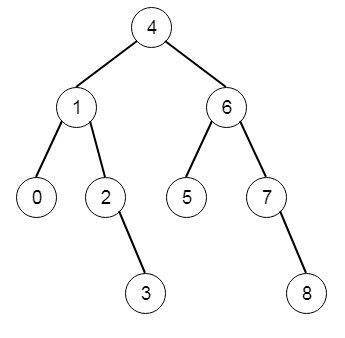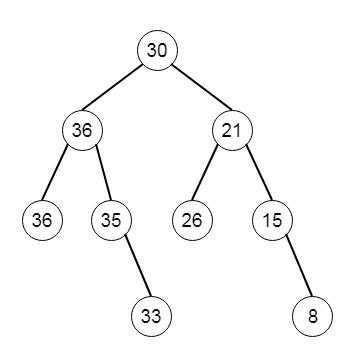# Binary Search Tree to Greater Sum Tree in C++

Suppose we have the root of a binary search tree with distinct values, we have to modify it so that every node has a new value equal to the sum of the values of the original tree that are greater than or equal to the value of node. We have to keep in mind that we are dealing with binary search tree, and this should maintain the properties of the BST. So if the input tree is like −Then the output tree will be −To solve this, we will follow these steps −

• set global := 0

• Define a recursive function solve(), that will take root as input.

• if right of root is not null, then solve(right of root)

• global := global + value of root

• if left of root is not null, then solve(left of root)

• return root

Let us see the following implementation to get better understanding −

## Example

Live Demo

#include <bits/stdc++.h>
using namespace std;
class TreeNode{
public:
int val;
TreeNode *left, *right;
TreeNode(int data){
val = data;
left = NULL;
right = NULL;
}
};
void insert(TreeNode **root, int val){
queue<TreeNode*> q;
q.push(*root);
while(q.size()){
TreeNode *temp = q.front();
q.pop();
if(!temp->left){
if(val != NULL)
temp->left = new TreeNode(val);
else
temp->left = new TreeNode(0);
return;
}else{
q.push(temp->left);
}
if(!temp->right){
if(val != NULL)
temp->right = new TreeNode(val);
else
temp->right = new TreeNode(0);
return;
}else{
q.push(temp->right);
}
}
}
TreeNode *make_tree(vector<int> v){
TreeNode *root = new TreeNode(v);
for(int i = 1; i<v.size(); i++){
insert(&root, v[i]);
}
return root;
}
void tree_level_trav(TreeNode*root){
if (root == NULL) return;
cout << "[";
queue<TreeNode *> q;
TreeNode *curr;
q.push(root);
q.push(NULL);
while (q.size() > 1) {
curr = q.front();
q.pop();
if (curr == NULL){
q.push(NULL);
}
else {
if(curr->left)
q.push(curr->left);
if(curr->right)
q.push(curr->right);
if(curr == NULL || curr->val == 0){
cout << "null" << ", ";
}else{
cout << curr->val << ", ";
}
}
}
cout << "]"<<endl;
}
class Solution {
public:
int global = 0;
TreeNode* bstToGst(TreeNode* root) {
if(root->right)bstToGst(root->right);
if(root->val != 0)
root->val = global = global + root->val;
if(root->left)bstToGst(root->left);
return root;
}
};
main(){
vector<int> v =
{4,1,6,1,2,5,7,NULL,NULL,NULL,3,NULL,NULL,NULL,8};
TreeNode *root = make_tree(v);
Solution ob;
tree_level_trav(ob.bstToGst(root));
}

## Input

[4,1,6,1,2,5,7,null,null,null,3,null,null,null,8]

## Output

[30, 36, 21, 37, 35, 26, 15, null, null, null, 33, null, null, null,
8, ]